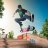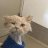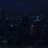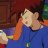# RSI and MACD study alert

D

#### drkellog

##### New member
VIP
I am trying to make a STUDY that will ring a bell when the RSI is below 30 and the MACD is above the zero line. Can anyone help me fix my code so it will RING. Its not ringing. A bonus would be if It could somehow plot it also but not necessary. Here is my code...

def Bearish = RSI("length" = 14, "over bought" = 70, "over sold" = 30)."RSI" is greater than or equal to 70 and MACD()."Value" is less than 0;

def Bullish =
RSI("length" = 14, "over bought" = 70, "over sold" = 30)."RSI" is less than or equal to 30 and MACD
()."Value" is greater than 0;Best Settings for MACD and RSI on 1 Minute Chart? Questions 2Add arrows to chart with MACD and RSI indicator? Questions 2SuperTrend Of MACD and AVG RSI Indicators 27RSI + MACD Confluence Indicator for ThinkorSwim Custom 18